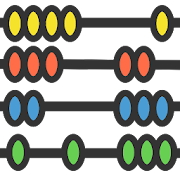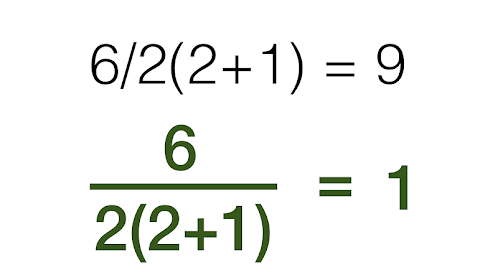MathSolver: Math calculator with solution & stepsEveryone
92
MathSolver is a free math app intended to help beginners, who struggle with basic math concepts. This app will show you step-by-step solution to all kinds of problem involving numbers in classroom teaching or everyday life. The app also serves as a math refresher for adults and homework helper for new learners.

* Arithmetic
- Subtraction with grouping and borrowing
- Long multiplication
- Long division method

* Order of operation
- Solve arithmetic expression with PEMDAS/ BODMAS rule.

* Factors and multiples
- LCM calculator
- GCF (HCF) calculator
- Prime factorization

* Fraction calculator
- Learn to simplify, add, subtract, multiply, divide and compare fractional numbers

*Basic Algebra (Solve for x)
- Linear equation in one variable
- Missing value in proportions

* Percentage calculator
- Learn how to work out a percentage

Math is easy and doable. Learn how to work with numbers with this math solver app.
Collapse

Review Policy
4.2
92 total
5
4
3
2
1

What's New

Collapse

Updated
September 18, 2018
Size
1.7M
Installs
10,000+
Current Version
1.2.9
Requires Android
4.0.3 and up
Content Rating
Everyone
Permissions
Offered By
Everyday Calculation Apps
Developer
Itanagar - 791111 Arunachal Pradesh India